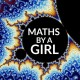# Forgotten Mathematicians: Egyptian Mathematics

Upon request, I have decided to continue this series on Forgotten Mathematicians.

In around 6000 BCE the ancient Egyptians settled along the Nile valley due to its fertile land. For both religious and agricultural reasons, they began to record the patterns of lunar phases and the seasons. In very early Egyptian history, the Pharaoh’s surveyors used their body parts to take measurements of the land and buildings (for example the cubit), and consequently a decimal numeral system was developed based on our ten fingers.

It is thought that the Egyptians introduced the earliest fully-developed base 10 numeration system – at least as early as 2700 BC. A stroke was used for units, a heel-bone symbol for tens, a coil rope for hundreds, etc.Source: ncetm.org.uk

The oldest mathematical text is thought to be the Moscow Papyrus, which dates to the 12th dynasty in 2000-1800 BC. The Moscow Papyrus and The Rhind Papyrus (dating from around 1650 BCE) contain mathematical problems, which are mostly practical, but a few are posed to teach the manipulation of the number system without a practical application. The Rhind Papyrus contains evidence of other mathematical knowledge such as unit fractions, composite and prime numbers, arithmetic, geometric and harmonic means and arithmetic and geometric series.

Furthermore, the ancient Egyptians were the first civilisation to develop and solve quadratic equations, as revealed by the Berlin Papyrus.

### Unit Fractions

Practical problems of trade and the market led to the development of fractions. They could also be used for the simple division sums.

For example is they needed to divide 3 loafs among 5 people:

• they would first divide 2 of the loaves into thirds;
• then divide third loaf into fifths;
• they would then divide the third that was left over into 5 pieces;
• Therefore, each person would get 1/3 + 1/5 + 1/15 = 3/5.

### Multiplication

This was achieved by the process of repeatedly multiplying the number in question and 1 by two on two separate sides. The corresponding numbers could then be used as a sort of multiplication reference table:

• the combination of powers of two which add up to the number to be multiplied was isolated;
• the corresponding numbers on the other side add up to give the answer.Sources: 1 | 2 | 3

Hope you enjoyed! M x

## 4 thoughts on “Forgotten Mathematicians: Egyptian Mathematics”

1.Joseph Nebus says:

I’ve always liked the Egyptian multiplication-by-doubling method. It seems to me like a good method for learning multiplication, too.

The unit fractions, now, that’s just astounding. I suppose with some experience one gets good at seeing how to make, say, 97/100ths out of a non-stupid set of reciprocals. But it’s hard to imagine getting to that point.

Liked by 1 person

1.mathsbyagirl says:

Yes, I agree. Their arithmetic ability is somewhat staggering and something quite admirable!

Like